# Equation of Motion for Two Objects

(This article was translated from Japanese to English using DeepL.)

We have previously learned how to formulate the equation of motion, a particularly important equation in mechanics.

https://www.yukimura-physics.com/entry/dyn-f22

By the way, there is not always only one object moving under a force.
Let’s consider the case where two objects move by exerting a force on each other!

## Formulate object-by-object

The explanation will be given while actually solving the questions.In this case, what is the equation of motion?
Here are some less desirable example first.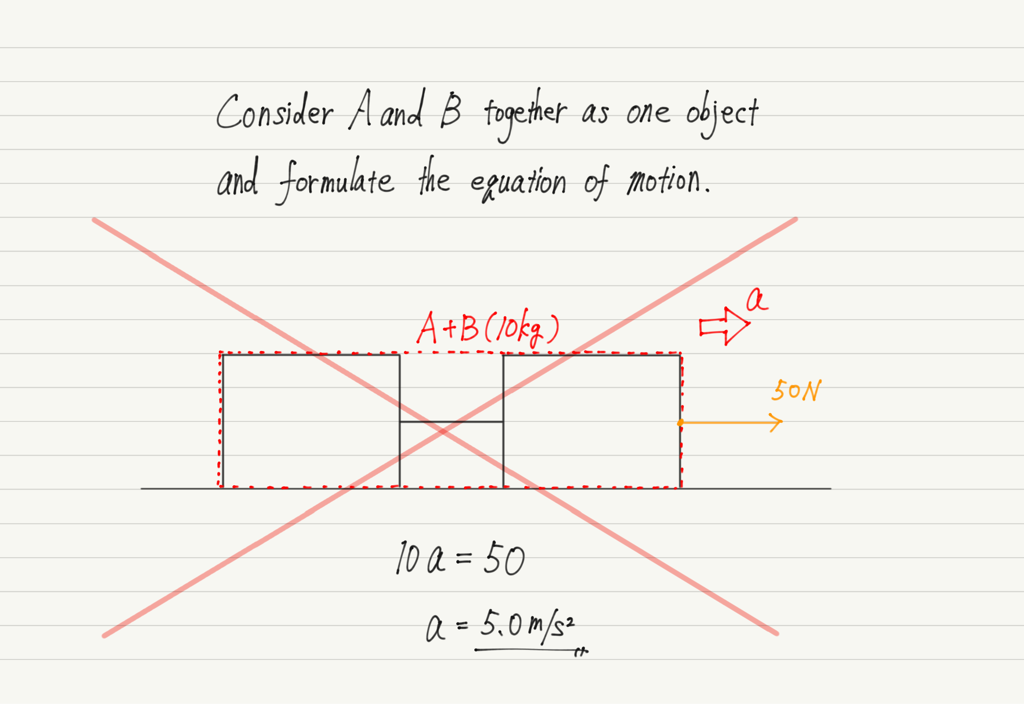It is not wrong to consider the two objects together as one object, but that often does not solve the problem (in the case of this problem, the tension force T cannot be obtained).

So what should we do?
Let’s formulate the equations of motion for each object separately!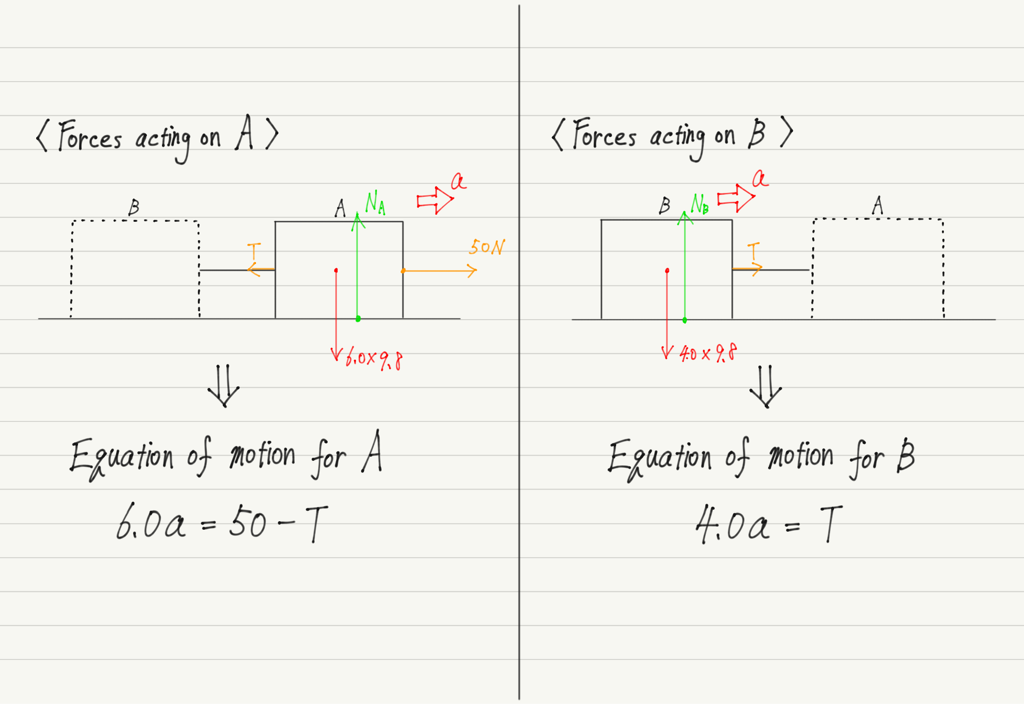The key is to draw a picture and distinguish the forces acting on each object.

This approach results in two equations of motion, but that is OK.
All that remains is to solve it. Since there are two equations, we are of course solving a simultaneous equation.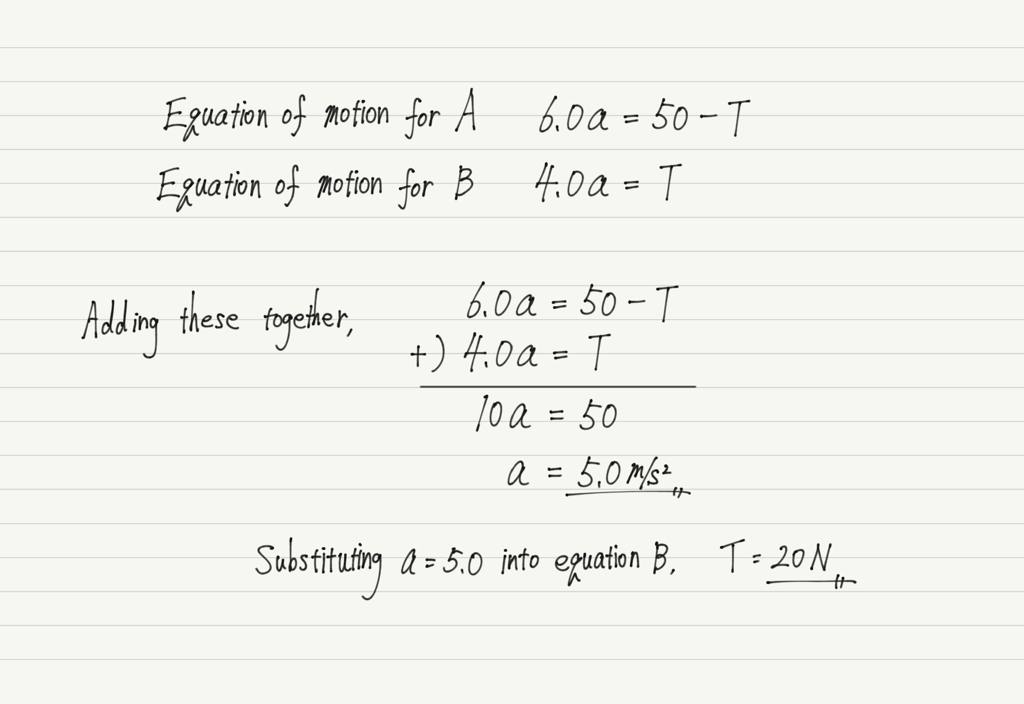Now that you know how to do it, let’s try one more question.

## Exercise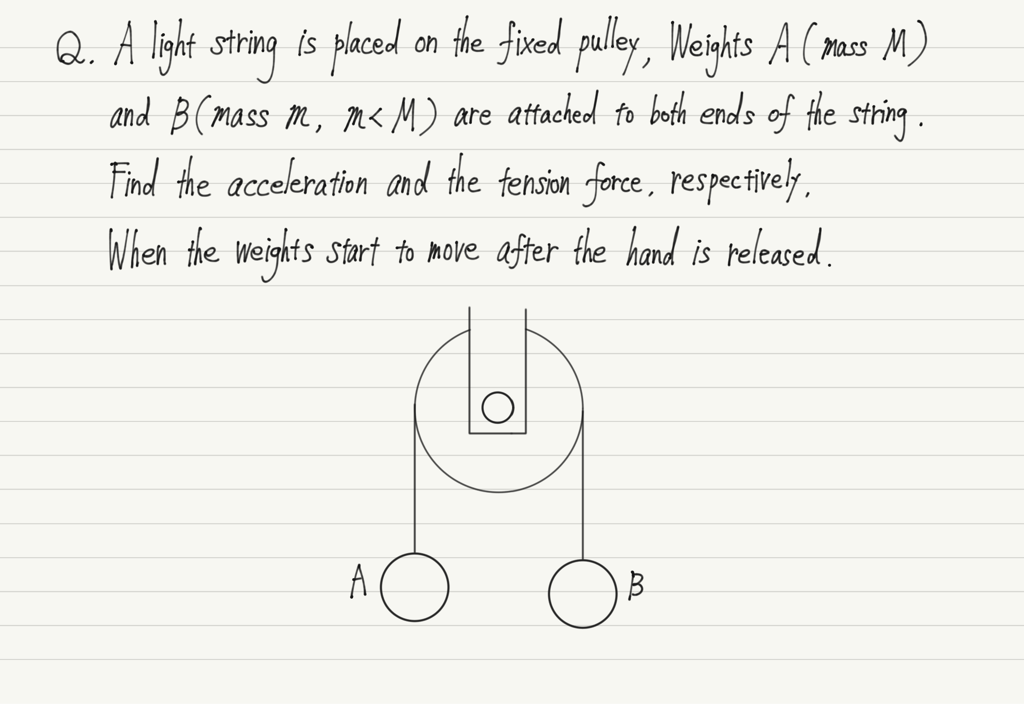Answers are below. Please scroll down when you have finished solving the question.

The point of this question is that the two objects have different directions of acceleration.
In these cases, assume the direction of acceleration for each object.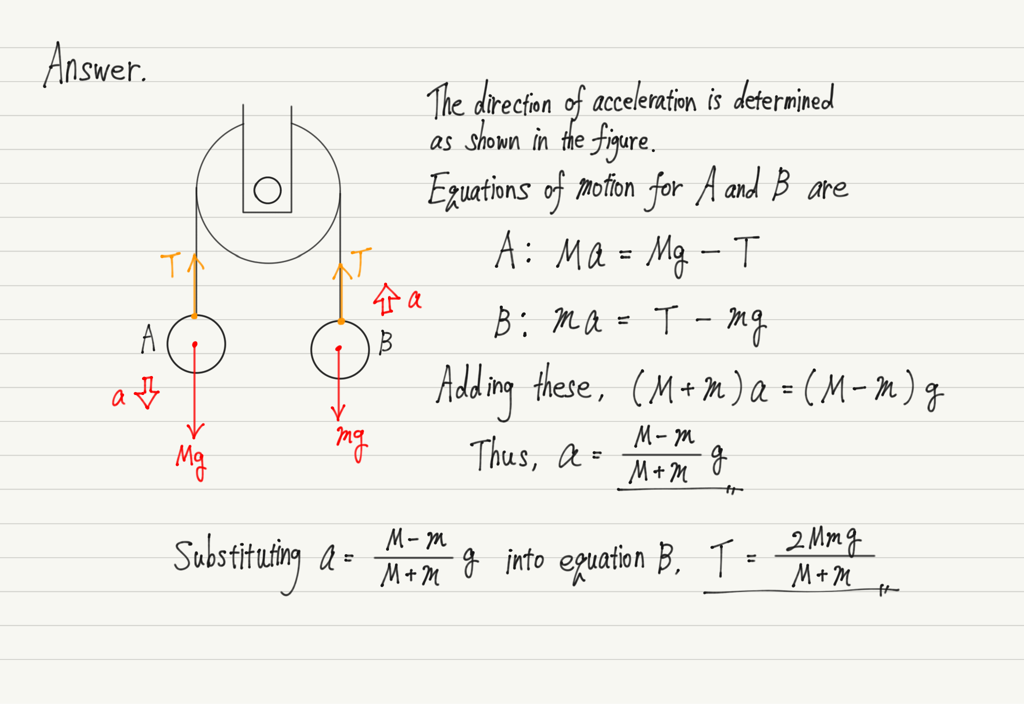The secret of the equation of motion is anyway to “formulate the equation of motion for each object”.

error: Content is protected !!